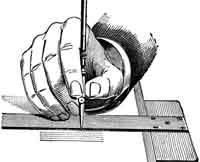#Difference Between Similar Terms and Objects

# Difference Betweeen ANOVA and RegressionANOVAÂ vs Regression

It is very difficult to distinguish the differences between ANOVA and regression. This is because both terms have more similarities than differences. It can be said that ANOVA and regression are the two sides of the same coin.

Both ANOVA (Analysis of Variance) and regression statistical models are only applicable if there is a continuous outcome variable. The regression model is based on one or more continuous predictor variables. On the contrary, the ANOVA model is based on one or more categorical predictor variables. ANOVA focuses on random variables, and regression focuses on fixed or independent or continuous variables. In ANOVA there can be several error terms whereas there is only a single error term in regression.

When ANOVA comes with three models, regression has mainly two models. Fixed effect, random effect, and mixed effect are the three models available with ANOVA. Multiple regression and linear regression are the more used models of regression. The initial test for identifying factors that influence a data set can be done by the ANOVA model. The test results from the ANOVA model can then be used in F-test on the relevance of the regression formula.

ANOVA is mainly used to determine if data from various groups have a common means or not. Regression is widely used for forecasting and predictions. It is also used for seeing which independent variable is related to the dependent variable. The first form of regression can be found in Legendre’s book ‘Method of Least Squares.’ It was Francis Galton who coined the term ‘regression’ in the 19th century.

ANOVA was first used informally by researchers in the 1800s. Sir Ronald Fisher in one of his articles formally used the term ANOVA in 1918. ANOVA got wide popularity after Fischer included this term in his book ‘Statistical Methods for Research Workers.’

Summary:

1.A regression model is based on one or more continuous predictor variables.

2.On the contrary, the ANOVA model is based on one or more categorical predictor variables.
3.In ANOVA there can be several error terms whereas there is only a single error term in regression.
4.ANOVA is mainly used to determine if data from various groups have a common means or not.

5.Regression is widely used for forecasting and predictions.

6.It is also used for seeing which independent variable is related to the dependent variable.
7.The first form of regression can be found in Legendre’s book ‘Method of Least Squares.’

8.It was Francis Galton who coined the term ‘regression’ in the 19th century.
9.ANOVA was first used informally by researchers in the 1800s. It got wide popularity after Fischer included this term in his book ‘Statistical Methods for Research Workers.’

### Search DifferenceBetween.net :

Custom Search

Help us improve. Rate this post!(3 votes, average: 2.67 out of 5)Loading...Email This Post : If you like this article or our site. Please spread the word. Share it with your friends/family.

### 1 Comment

1. its really understandable ans thanks buddy

Please note: comment moderation is enabled and may delay your comment. There is no need to resubmit your comment.

Articles on DifferenceBetween.net are general information, and are not intended to substitute for professional advice. The information is "AS IS", "WITH ALL FAULTS". User assumes all risk of use, damage, or injury. You agree that we have no liability for any damages.